### Home > PC > Chapter 6 > Lesson 6.2.2 > Problem6-89

6-89.
1. Solve for x on the indicated domain. Homework Help ✎

1. cos x = 0, [0, 2π]

2. 2 sin x +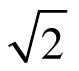= 0, [0, 2π]

3. cos x(2 sin x +) = 0, [0, 2π]

4. cos x(2 sin x +) = 0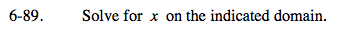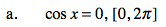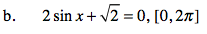$\text{sin }x=-\frac{\sqrt{2}}{2}$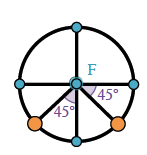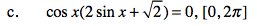Use the Zero Product Property. Then combine parts (a) and (b).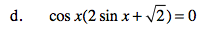Solve for all solutions.
Add a 2πn to each solution in [0,2π] where n is any integer.